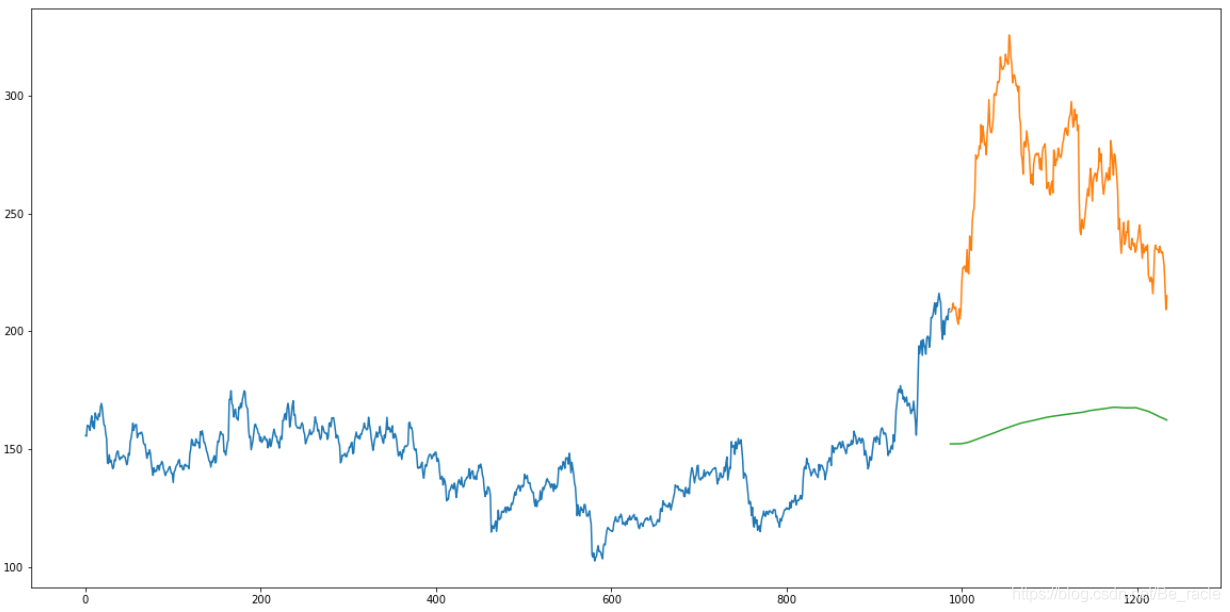# 时间序列预测中的机器学习方法（一）：移动平均（Moving Average）

## 2.数据集

``````import pandas as pd
import numpy as np
import matplotlib.pyplot as plt

#setting figure size
from matplotlib.pylab import rcParams
rcParams['figure.figsize'] = 20,10

#for normalizing data
from sklearn.preprocessing import MinMaxScaler
scaler = MinMaxScaler(feature_range=(0, 1))
``````

``````df = pd.read_csv('NSE-TATAGLOBAL11.csv')
``````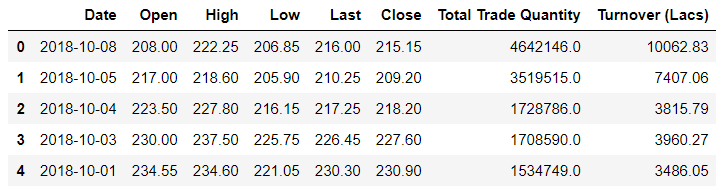• Open和Close代表该股票在某一天的交易开始和最终的价格。
• High，Low和Last代表当天股票的最高价，最低价和最后价。

``````#setting index as date
df['Date'] = pd.to_datetime(df.Date, format = '%Y-%m-%d')
df.index = df['Date']
``````

``````plt.figure(figsize=(16,8))
plt.plot(df['Close'], label='Close Price history')
``````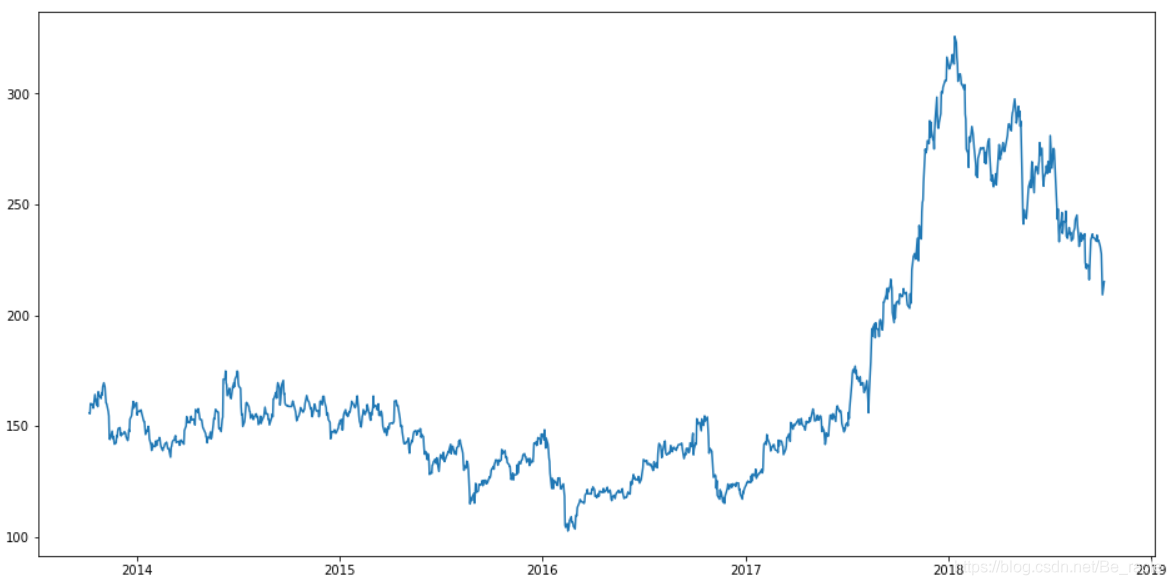## 3.移动平均(Moving Average)

“平均”是我们日常生活中最常见的事物之一。例如，计算平均分数以确定总体性能，或者找到过去几天的平均温度以了解当前的温度。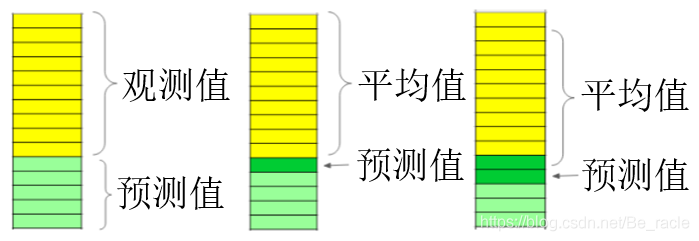``````#creating dataframe with date and the target variable
data = df.sort_index(ascending=True, axis=0)
new_data = pd.DataFrame(index=range(0,len(df)),columns=['Date', 'Close'])

for i in range(0,len(data)):
new_data['Date'][i] = data['Date'][i]
new_data['Close'][i] = data['Close'][i]

new_data
``````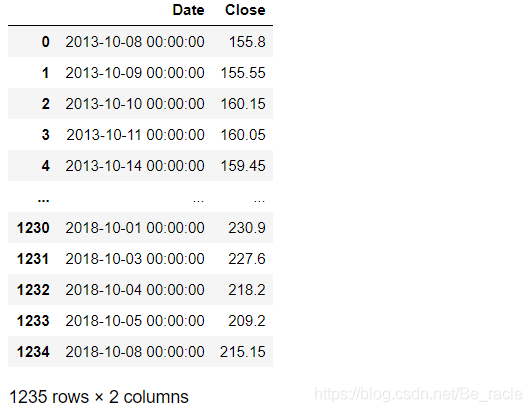``````# splitting into train and validation
train = new_data[:987]
valid = new_data[987:]

print('Shape of training set:')
print(train.shape)
print('Shape of validation set:')
print(valid.shape)
``````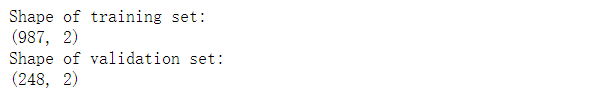``````# making predictions
preds = [] #移动平均求出的预测集
for i in range(0,valid.shape):
a = train['Close'][len(train)-248+i:].sum() + sum(preds) #从739开始往后做移动平均
b = a/248 #移动窗口设置为248
preds.append(b)
``````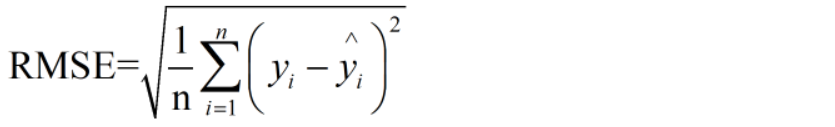``````# checking the results (RMSE value)
# 比如RMSE=10，可以认为回归效果相比真实值平均相差10
rms=np.sqrt(np.mean(np.power((np.array(valid['Close'])-preds),2)))
print('RMSE value on validation set:')
print(rms)
``````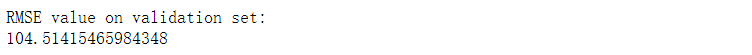``````valid['Predictions'] = 0
valid['Predictions'] = preds
plt.plot(train['Close'])
plt.plot(valid[['Close', 'Predictions']])
``````